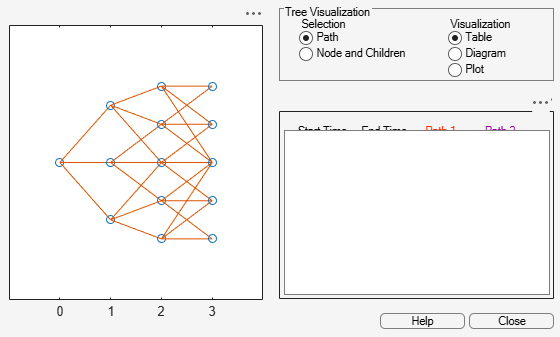Main Content

# trintreeshape

Shape of recombining trinomial tree

## Syntax

``[NumLevels,NumPos,NumStates] = treeshape(TrinTree)``

## Description

example

````[NumLevels,NumPos,NumStates] = treeshape(TrinTree)` returns information on a recombining trinomial tree's shape.```

## Examples

collapse all

This example shows how to obtain information on a recombining trinomial tree's shape.

Create a `HW` tree by loading the example file.

`load deriv.mat; `

With `treeviewer` you can see the general shape of the `HW` interest-rate tree.

`treeviewer(HWTree)`Use `trintreeshape` to display the shape of the binomial tree.

`[NumLevels, NumPos, IsPriceTree] = trintreeshape(HWTree) `
```NumLevels = 4 ```
```NumPos = 1×4 1 1 1 1 ```
```IsPriceTree = 1×4 1 3 5 5 ```

## Input Arguments

collapse all

Recombining price or interest-rate trinomial tree, specified as a struct that is created using one of the following functions:

Data Types: `struct`

## Output Arguments

collapse all

Number of time levels of tree, returned as a numeric.

Length of the state vectors in each level, returned as a `1`-by-`NUMLEVELS` vector.

Number of state vectors in each level, returned as a `1`-by-`NUMLEVELS` vector.

## See Also

### Topics

Introduced before R2006a

Download ebook